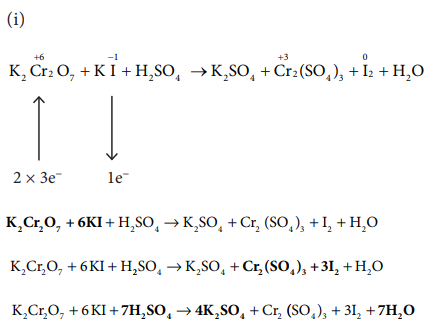Home | | Chemistry 11th std | Solved Example Problems: Basic Concepts of Chemistry and Chemical Calculations

# Solved Example Problems: Basic Concepts of Chemistry and Chemical Calculations

Chemistry : Basic Concepts of Chemistry and Chemical Calculations - Solved Example Problems

32) The density of carbon dioxide is equal to 1.965 kgm-3 at 273 K and 1 atm pressure. Calculate the molar mass of CO2.

Given :

The density of CO2 at 273 K and 1 atm pressure = 1.965 kgm-3

Molar mass of CO2 = ?

At 273 K and 1 atm pressure, 1 mole of CO2 occupies a volume of 22.4 LMolar mass of CO2 = 44 gmol–1

33) Which contains the greatest number of moles of oxygen atoms

i) 1 mol of ethanol

ii) 1 mol of formic acid

iii) 1 mol of H2O34) Calculate the average atomic mass of naturally occurring magnesium using the following data35) In a reaction x + y + z2  xyz2 identify the Limiting reagent if any, in the following reaction mixtures.

(a) 200 atoms of x + 200 atoms of y + 50 molecules of z2

(b) 1mol of x + 1 mol of y+3 mol of z2

(c) 50 atoms of x + 25 atoms of y+50 molecules of z2

(d) 2.5 mol of x +5 mol of y+5 mol of z2

Reaction : x + y + z2  xyz236) Mass of one atom of an element is 6.645 x 10-23 g. How many moles of element are there in 0.320 kg.

Given: mass of one atom = 6.645 × 10–23 g

mass of 1 mole of atom = 6.645 × 10–23 g × 6.022 × 1023

= 40g= 8 mol.

37) What is the difference between molecular mass and molar mass? Calculate the molecular mass and molar mass for carbon monoxide.

38) What is the empirical formula of the following ?

i) Fructose (C6H12O6) found in honey

ii) Caffeine (C8H10N4O2) a substance found in tea and coffee.39) The reaction between aluminium and ferric oxide can generate temperatures up to 3273 K and is used in welding metals. (Atomic mass of AC = 27 u Atomic mass of 0 = 16 u)

2Al + Fe2O3  Al2O3 +2Fe; If, in this process, 324 g of aluminium is allowed to react with 1.12 kg of ferric oxide.

i) Calculate the mass of Al2O3 formed.

ii) How much of the excess reagent is left at the end of the reaction?40) How many moles of ethane is required to produce 44 g of CO2 (g) after combustion.41) Hydrogen peroxide is an oxidising agent. It oxidises ferrous ion to ferric ion and reduced itself to water. Write a balanced equation.1 H2O2 + 2Fe2+  Fe3+ + H2O

H2O2 + 2Fe2+ + 2H+  2Fe3+ + 2H2O

42) Calculate the empirical and molecular formula of a compound containing 76.6% carbon, 6.38 % hydrogen and rest oxygen its vapour density is 47.43) A Compound on analysis gave Na = 14.31% S = 9.97% H= 6.22% and O= 69.5% calculate the molecular formula of the compound if all the hydrogen in the compound is present in combination with oxygen as water of crystallization. (molecular mass of the compound is 322).Molecular formula = Na2 S H20 O14

Since all the hydrogen in the compound present as water

Molecular formula is Na2 SO4 . 10H2O

44) Balance the following equations by oxidation number method

i) K2Cr2O7 + KI + H2SO4  K2SO4 + Cr2(SO4)3 +I2+H2O

ii) KMnO4 + Na2SO3  MnO2 + Na2SO4 + KOH

iii) Cu+ HNO3  Cu(NO3)2 + NO2+ H2O

iv) KMnO4+H2C2O4 + H2SO4  K2SO4 + MnSO4 + CO2 + H2O45) Balance the following equations by ion electron method.

i) KMnO4 + SnCl2+HCl  MnCl2 + SnCl4 + H2O + KCl

ii) C2O42_ + Cr2 O72_  Cr3+ + CO2 (in acid medium)

iii) Na2S2O3 + I2  Na2S4O6 + NaI ( in acid medium)

iv) Zn +NO3_  Zn2+ + NO